## How to Convert Angles from Radians to Degrees in Excel

This post will guide you how to convert angles from radians to degrees with a formula in Excel. How do I convert angle between radians to degrees in Excel.

When you want to solve trigonometric expressions like sine, cosine and tangent, you need to use radians to perform these calculations. So if your have a range of list that contain degree values, and you have to convert it to radians for calculation.

You may be have a list of data that contain radian values, and you want to convert it from radians to degrees. You can use a build-in function known as RADIANS function, it can be used to convert degrees to radians. And you can use the DEGREES function to convert radians into degrees in Excel.

## Convert angles from Radians to degrees

Assuming that you have a list of degrees in range B1:B4, and you want to convert those values into radians, just use the following formula:

`=RADIANS(B1)`

Type this formula into a blank cell or the adjacent cell C1, press Enter key to apply this formula. And then drag the AutoFill Handle down to other cells until to C4.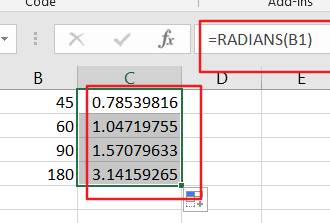You would see that all degree values has been converted to radian values.

## Convert Angles from Degrees to Radians

If you want to convert radian values to degree values, just use the below formula based on the DEGREES function.

`=DEGREEES(C1)`

Type this formula into a blank cell or the adjacent cell D1, press Enter key to apply this formula. And then drag the AutoFill Handle down to other cells until to D4.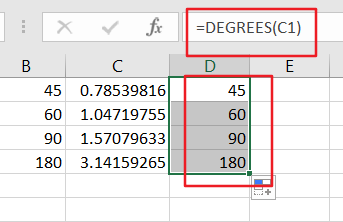You would see that all radian values have been converted to degree values.

### Related Functions

• Excel DEGREES Function
The Excel DEGREES function converts radians into degrees. And it will return a numeric value.The syntax of the DEGREES function is as below:=DEGREES (angle)…

## Excel DEGREES Function

This post will guide you how to use Excel DEGREES function with syntax and examples in Microsoft excel.

### Description

The Excel DEGREES function converts radians into degrees. And it will return a numeric value. For examples, if you pass the PI() function into the DEGREES function as its argument, and it will return 180.

The DEGREES function is a build-in function in Microsoft Excel and it is categorized as a Math and Trigonometry Function.

The DEGREES function in Excel 2016, Excel 2013, Excel 2010, Excel 2007, Excel 2003, Excel XP, Excel 2000, Excel 2011 for Mac.

### Syntax

The syntax of the DEGREES function is as below:

`=DEGREES (angle)`

Where the DEGREES function arguments is:

• angle -This is a required argument. .The angle in radians that you want to convert to degrees.

### Excel DEGREES Function Examples

The below examples will show you how to use Excel DEGREES Function to convert radians into degrees.

1# get the degrees of pi radians, enter the following formula in Cell B1.

`=DEGREES (PI())`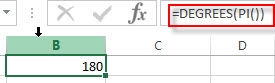2# get the degrees of 1.25 radians, enter the following formula in Cell B2.

`=DEGREES (1.25)`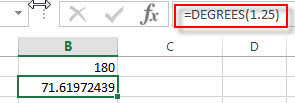### Related Functions

• Excel PI function
The Excel PI function returns the value of mathematical constant PI. The returned value is 3.14159265358979 and ti will accurate to 15 digits.The syntax of the PI function is as below:=PI()…

## Excel ATAN2 Function

This post will guide you how to use Excel ATAN2 function with syntax and examples in Microsoft excel.

### Description

The Excel ATAN2 function returns the arctangent, or inverse tangent, of the specified x- and y-coordinates. And you can use the ATAN2 function to calculate the arctangent of a given x and y coordinates. The returned value is an angle between –pi/2 and pi/2.

The ATAN2 function is a build-in function in Microsoft Excel and it is categorized as a Math and Trigonometry Function.

The ATAN2 function is available in Excel 2016, Excel 2013, Excel 2010, Excel 2007, Excel 2003, Excel XP, Excel 2000, Excel 2011 for Mac.

### Syntax

The syntax of the ATAN2 function is as below:

`= ATAN2 (x_num, y_num)`

Where the ATAN2 function arguments are:

• x_num -This is a required argument. The x-coordinate of a point.
• Y_num – This is a required argument. The y-coordinate of a point.

Note:

• If the values of both x_num and y_num arguments are 0, the ATAN2 will return the #DIV/0! Error.
• The returned value of ATAN2(a,b) is equal to the formula ATAN2(b,a).
• If you want to express the arctangent in degrees, you just need to multiply the result by 180/PI() or you can use the DEGREES function.
• If the supplied number is not a numeric value, the ATAN function will get the #VALUE！ Error。
• If the returned result is a positive value, it indicates that it is a counterclockwise angle from the x-axis. If the returned result is a negative value, it indicates that it is a clockwise angle.

### Excel ATAN2Function Examples

The below examples will show you how to use Excel ATAN2 Function to return the arctangent of x and y coordinates.

1# get the arctangent of the x- and y-coordinates 2,2 in radians, enter the following formula in Cell B1.

`=ATAN2(2,2)`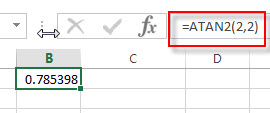The returned result is 0.785398.

2# get the arctangent of the x- and y-coordinates -2,-2 in radians, -3*pi/4, using the following formula in Cell B2.

`=ATAN2(-2,-2)`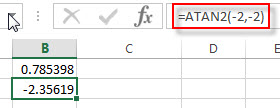This formula will return the value -2.35619.

3# get the arctangent of the x- and y-coordinates 2,2 in degrees, using the following formula in Cell B3.

`=ATAN2(2,2)*180/PI()`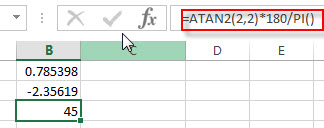Or you can use the below formula to achieve the same result:

`=DEGREES(ATAN2(2.2))`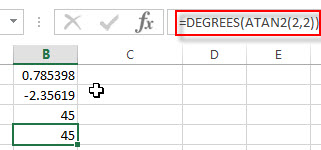### Related Functions

• Excel ATAN function
The Excel ATAN function returns the arctangent of a number. So this function can be used to calculates the arctangent of a supplied number. The returned angle is given in radians in the range –pi/2 to pi/2. The syntax of the ATAN function is as below:= ATAN (number)…
• Excel ATANH function
The Excel ATANH function returns the inverse hyperbolic tangent of a given number. The syntax of the ATANH function is as below:= ATANH (number)…
• Excel ACOS function
The Excel ACOS function returns the arccosine value of a number.The ACOS function is a build-in function in Microsoft Excel and it is categorized as a Math and Trigonometry Function.The syntax of the ACOS function is as below:= ACOS(number)…
• Excel ACOSH function
The Excel ACOSH function returns the inverse hyperbolic cosine of a number.The syntax of the ACOSH function is as below:= ACOSH (number)…
• Excel ASIN function
The Excel ASIN function returns the arcsine value of a number.The syntax of the ASIN function is as below:= ASIN (number)…
• Excel DEGREES Function
The Excel DEGREES function converts radians into degrees. And it will return a numeric value. The syntax of the DEGREES function is as below:=DEGREES (angle)…

## Excel ATAN Function

This post will guide you how to use Excel ATAN function with syntax and examples in Microsoft excel.

### Description

The Excel ATAN function returns the arctangent of a number. So this function can be used to calculates the arctangent of a supplied number. The returned angle is given in radians in the range –pi/2 to pi/2. Or the returned value is a numeric value.

The ATAN function is a build-in function in Microsoft Excel and it is categorized as a Math and Trigonometry Function.

The ATAN function is available in Excel 2016, Excel 2013, Excel 2010, Excel 2007, Excel 2003, Excel XP, Excel 2000, Excel 2011 for Mac.

### Syntax

The syntax of the ATAN function is as below:

`= ATAN (number)`

Where the ATAN function arguments is:

• number -This is a required argument. The tangent of the angle that you want to get.

Note:

• If you want to express the arctangent in degrees, you just need to multiply the result by 180/PI() or you can use the DEGREES function.
• If the supplied number is not a numeric value, the ATAN function will get the #VALUE！ Error。

### Excel ATAN Function Examples

The below examples will show you how to use Excel ATAN Function to return the arctangent for a given number.

1# get the arctangent of 2 in radians, pi/4, enter the following formula in Cell B1.

=ATAN(2)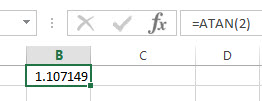The returned result is 1.107149.

2# get the arctangent of 2 in degrees, using the following formula in Cell B2.

`=ATAN(2)* 180/PI()`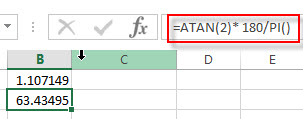This formula will return the value 63.43495.

Or you can use the below formula to achieve the same result:

`=DEGREES(ATAN(2))`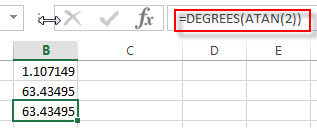### Related Functions

• Excel PI function
The Excel PI function returns the value of mathematical constant PI. The returned value is 3.14159265358979 and ti will accurate to 15 digits.The syntax of the PI function is as below:=PI()…
• Excel ATAN2 function
The Excel ATAN2 function returns the arctangent, or inverse tangent, of the specified x- and y-coordinates. And you can use the ATAN2 function to calculate the arctangent of a given x and y coordinates. The syntax of the ATAN2 function is as below:= ATAN2 (x_num, y_num)…
• Excel ATANH function
The Excel ATANH function returns the inverse hyperbolic tangent of a given number. The syntax of the ATANH function is as below:= ATANH (number)…
• Excel ACOS function
The Excel ACOS function returns the arccosine value of a number.The ACOS function is a build-in function in Microsoft Excel and it is categorized as a Math and Trigonometry Function.The syntax of the ACOS function is as below:= ACOS(number)…
• Excel ACOSH function
The Excel ACOSH function returns the inverse hyperbolic cosine of a number.The syntax of the ACOSH function is as below:= ACOSH (number)…
• Excel ASIN function
The Excel ASIN function returns the arcsine value of a number.The syntax of the ASIN function is as below:= ASIN (number)…
• Excel ASIN function
The Excel ATAN function returns the arctangent of a number. So this function can be used to calculates the arctangent of a supplied number. The returned angle is given in radians in the range –pi/2 to pi/2. The syntax of the ATAN function is as below:= ATAN (number)…
• Excel DEGREES Function
The Excel DEGREES function converts radians into degrees. And it will return a numeric value. The syntax of the DEGREES function is as below:=DEGREES (angle)…